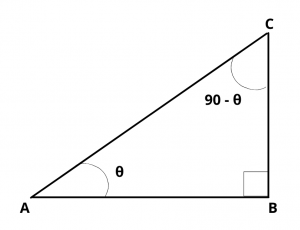# Prove that $$Tan(90 – \theta)$$ = $$Cot\theta$$.

## Solution :

Draw a right angled triangle ABC right angled at B.Let $$\angle$$ A = $$\theta$$,  then $$\angle$$ C = 90 – $$\theta$$

cot A = $$cot\theta$$ = $$AB\over BC$$                     ……..(1)

tan C = $$tan(90 – \theta)$$ = $$AB\over BC$$             ……..(2)

From (1) and (2), we have

$$tan(90 – \theta)$$ = $$cot\theta$$